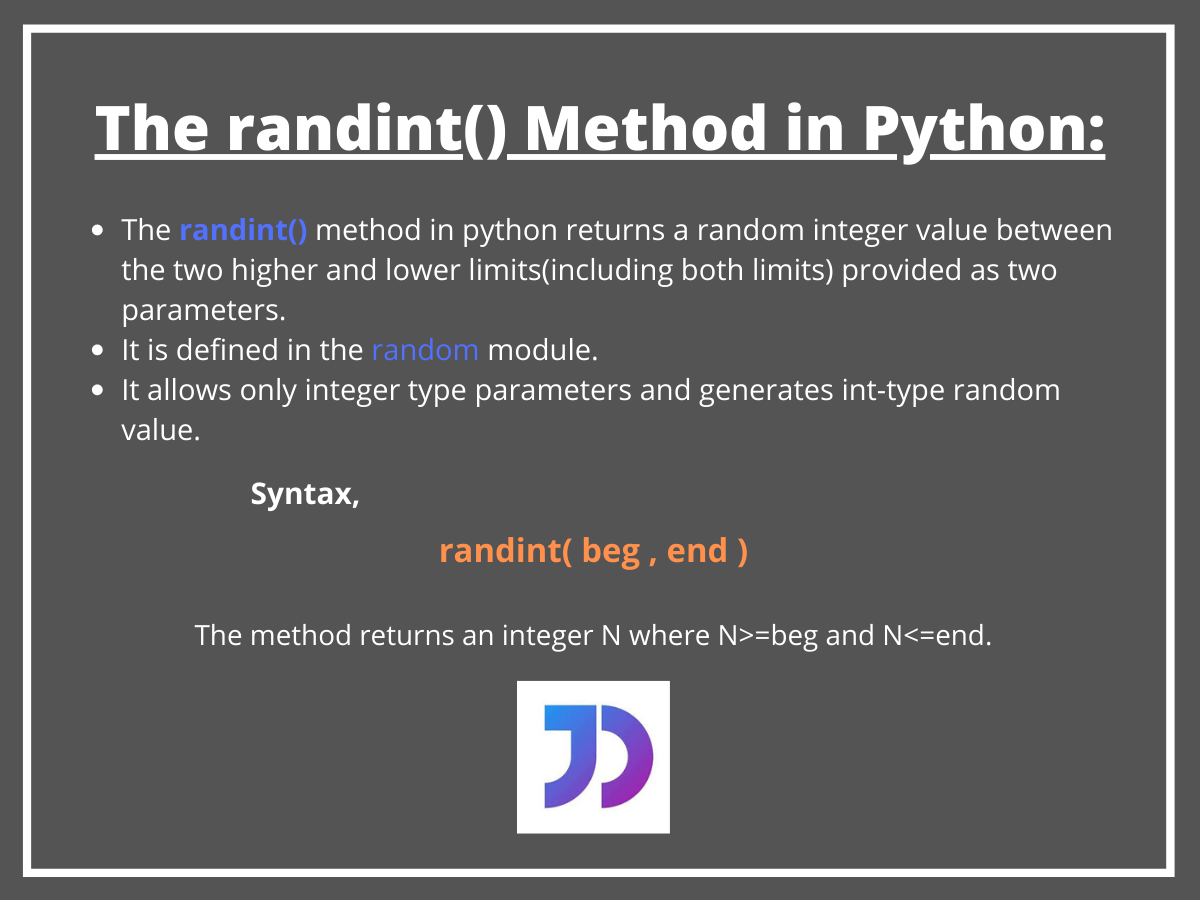# The randint() Method in Python

Published on August 3, 2022By SnehWhile we believe that this content benefits our community, we have not yet thoroughly reviewed it. If you have any suggestions for improvements, please let us know by clicking the “report an issue“ button at the bottom of the tutorial.

## Introduction

In this tutorial, we are going to focus on the `randint()` method in Python. In our previous tutorials, we saw different random number generating methods defined inside the random module in our Random Number Tutorial in Python.

So, as you already know, we need to import the random module in Python first to begin using the randint() method. The module essentially creates pseudo-randomness.

## The randint() method Syntax

Basically, the `randint()` method in Python returns a random integer value between the two lower and higher limits (including both limits) provided as two parameters.

It should be noted that this method is only capable of generating integer-type random value. Take a look at the syntax so that we can further incorporate the method.

``````#randint() Syntax
randint(lower limit , upper limit)
``````

Here,

• lower limit is the starting point from and including which the random integer would be generated,
• uppwer limit is the stopping point up to which the method would return the random integer.

The above example returns an integer N where N>=beg and N<=end.

It works in the same way `randrange(beg,end)` does, and hence is an alias for the same.

## The randint() Method Example

Let us look at the given code below, it illustrates the use and working of the `randint()` method.

``````import random
beg=10
end=100
random_integer = random.randint(beg, end)
print("The random integer is :", random_integer)
``````

Output:

Clearly, we can see that the `randint()` method generates a random integer value within the limit 1-100.

Is this value random? What happens when we call the method multiple times? Does it return the same value?

## Multiple randint() Method Call

The code snippet below answers all the above-mentioned questions and gives us a clear understanding.

``````import random
beg=10
end=100
for i in range(5):
print(random.randint(beg, end))
``````

Output:

For the above code, repeating the `random.randint()` method gives us different random integers for each call within the limit 10 to 100.

Hence, we can infer that the values are random for each call and do not overlap in our case. Furthermore, when the number of calls is large and the range is quite smaller, in that case, the random values generated may collide or overlap.

As said earlier, one must ensure that the higher and lower limit parameters have to be an integer type. For other types, we get a ValueError as shown below.

``````import random
beg=5.3
end=10.2
print(random.randint(beg, end))
``````

Output:

``````Traceback (most recent call last):
File "C:/Users/sneha/Desktop/test.py", line 4, in <module>
print(random.randint(beg, end))
File "C:\Users\sneha\AppData\Local\Programs\Python\Python37-32\lib\random.py", line 222, in randint
return self.randrange(a, b+1)
File "C:\Users\sneha\AppData\Local\Programs\Python\Python37-32\lib\random.py", line 186, in randrange
raise ValueError("non-integer arg 1 for randrange()")
ValueError: non-integer arg 1 for randrange()

Process finished with exit code 1
``````

## Conclusion

I hope this brief tutorial on the randint() method in Python has made the function clear for you. Your feedback is always welcome through the comments.

Click below to sign up and get \$200 of credit to try our products over 60 days!

### Popular Topics##### Hollie's Hub for Good

Working on improving health and education, reducing inequality, and spurring economic growth? We’d like to help.##### Become a contributor

You get paid; we donate to tech nonprofits.

##### Welcome to the developer cloud

DigitalOcean makes it simple to launch in the cloud and scale up as you grow – whether you’re running one virtual machine or ten thousand.© 2023 DigitalOcean, LLC.